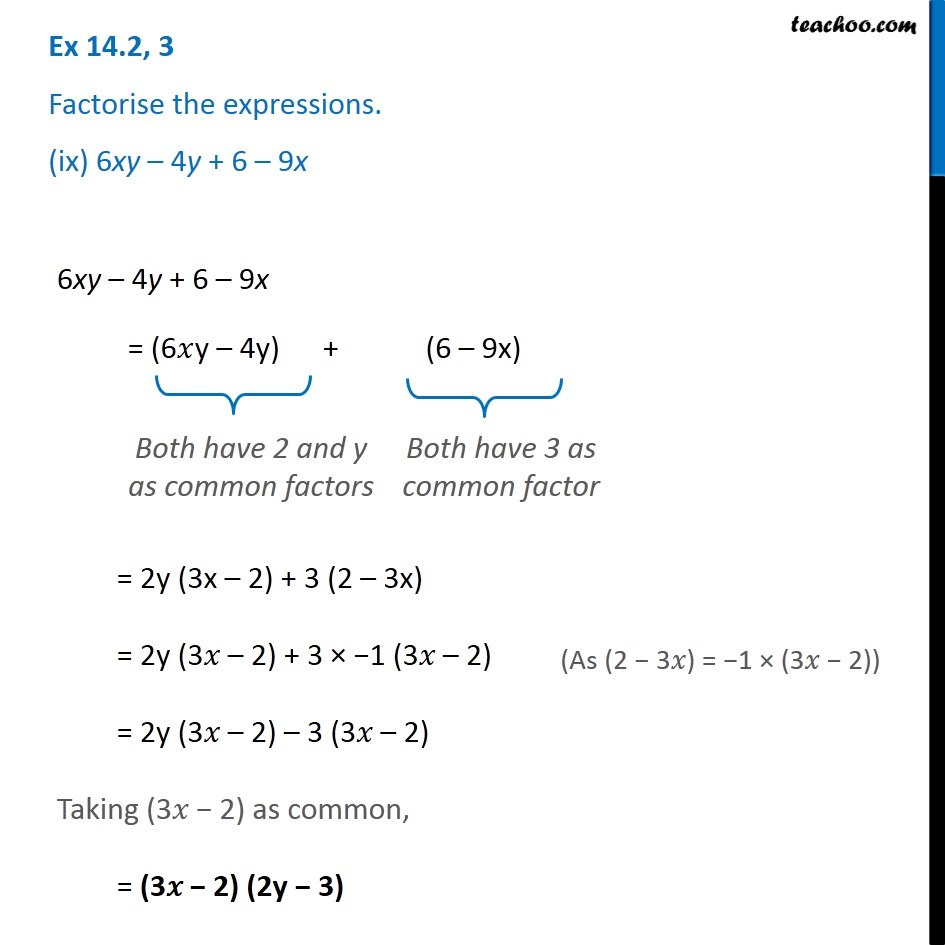1. Chapter 14 Class 8 Factorisation
2. Serial order wise
3. Ex 14.2

Transcript

Ex 14.2, 3 Factorise the expressions. (ix) 6xy – 4y + 6 – 9x 6xy – 4y + 6 – 9x = (6𝑥y – 4y) + (6 – 9x) Both have 2 and y as common factors Both have 3 as common factor = 2y (3x – 2) + 3 (2 – 3x) = 2y (3𝑥 – 2) + 3 × −1 (3𝑥 – 2) = 2y (3𝑥 – 2) – 3 (3𝑥 – 2) Taking (3𝑥 − 2) as common, = (3𝑥 − 2) (2y − 3) (As (2 − 3𝑥) = −1 × (3𝑥 − 2))

Ex 14.2